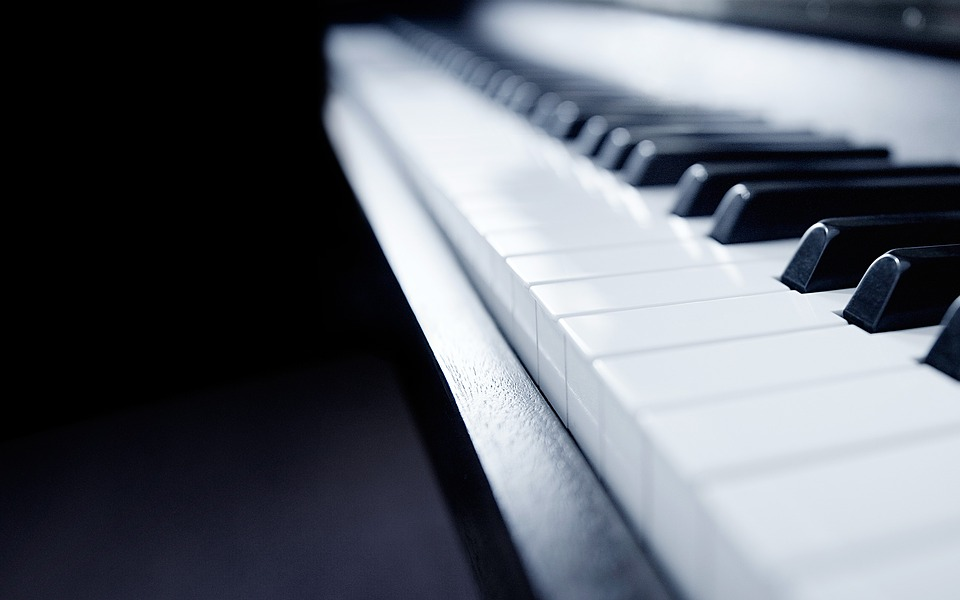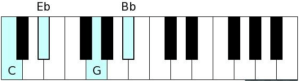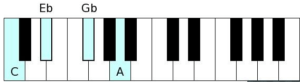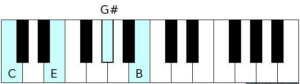# PIANO CHORDS (3): Seventh Piano ChordsNow that you’ve gotten a comprehensive look at how to form intervals, scales, and foundational  chords, let’s take it a step further by expanding our chords. If you are new in this series. Check out the previous lessons.. PIANO CHORDS 1: THE FOUNDATION OF CHORDS    OR PIANO CHORDS 2: DIMINISHED AND AUGMENTED CHORDS

We will be studying the below 7th chords but in this lesson, we will discuss the first four

• Major seventh chord
• Minor seventh chord
• Diminished seventh chord
• Augmented major seventh chord
• Half­-Diminished seventh chord
• Dominant seventh chord
• Augmented seventh chord
• Minor-­major seventh chord

Chord Type:​Major seventh chord

Scale Formula:​1+3+5+7

Interval Formula:​major third + minor third + major third

Notes in C:​ C + E + G + B

C + (major third) = C + E

E + (minor third) = E + G

G + (major third) = G + B

Combine all intervals: C + E + G + BChord Type:​ Minor seventh chord

Scale Formula:​  1+♭3+5+♭7

Interval Formula:​ minor third + major third + minor third

Notes in C:​ C + Eb + G + Bb

C + (minor third) = C + Eb

Eb + (major third) = Eb + G

G + (minor third) = G + Bb

Combine all intervals: C + Eb + G + BbIf you are having issues with understanding Intervals, you can read our lessons on Intervals.. here

Chord Type:​ Diminished seventh chord

Scale Formula:​  1+♭3+♭5+♭♭7

Interval Formula:​ minor third + minor third + minor third

Notes in C:​ C + Eb + Gb + Bbb

*Don’t let the ♭♭ (double flat) throw you off. If one ♭ means to lower by one half step, then ♭♭  must mean to lower by how many half steps? You got it, 2. (And because 2 half steps are equal to 1 whole step, you can think of this as one whole step as well). In other words, this formula could really be

1 + ♭3 + b5 + 6 (although saying “A” instead of “Bbb” would not be correct for generic

interval  purposes. You can read our posts on intervals here

C + (minor third) = C + Eb

Eb + (minor third) = Eb + Gb

Gb + (minor third) = Gb + Bbb (aka – “A”)

*As funny as it sounds, my piano graphic script does not do “Bbb” so I’m forced to place “A” there.  But you know the truth!!!

Combine all intervals: C + Eb + Gb + Bbb (aka – “A”)Chord Type:​Augmented major seventh chord

Scale Formula:​1+3+#5+7

Interval Formula:​major third + major third + minor third

Notes in C:​C + E + G# + B

C + (major third) = C + E

E + (major third) = E + G#

G# + (minor third) = G# + B

Combine all intervals: C + E + G# + BIn our next lesson, we will continue on the other 7th Chords. Feel free to drop your questions…###### Daniel

Olowu Daniel is a Musicologist and Pianist that started his musical career as a Pianist in the year 2007. He is a Music Tutor also. feel free to contact me for anything. +2348176251802..I am the Crazy Music Freak you've not heard about.